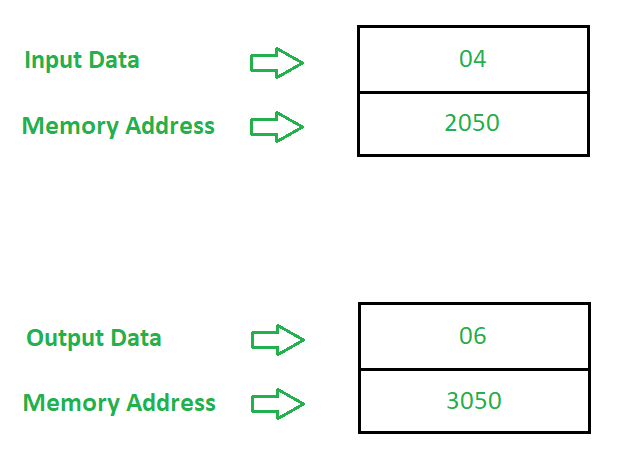# 8085 program to convert binary numbers to gray

Prerequisite – Binary to/from Gray Code Problem – Write an assembly language program in 8085 microprocessor to convert binary numbers to gray. Example –Algorithm –

1. Set the Carry Flag (CY) to 0.
3. Move the data of A(accumulator) into register B.
4. Rotate the bits of A to right.
5. XOR the contents of register A and B.
6. Store the result at memory address 3050.
7. Stop.

Program –

Explanation –

1. STC is used to set carry flag (CY) to 1.
2. CMC is used to take 1’s compliment of the contents of carry flag (CY).
3. LDA 2050 is used load the data from address 2050 in A.
4. MOV B, A is used to move the data of A into B.
5. RAR is used to rotate the bits of A along with carry flag (CY) to right one time.
6. XRA B is used to perform XOR operation between the contents of register A and B.
7. STA 3050 is used to store the contents of A to 3050.
8. HLT is used end the program.

Reduced bit transitions: Gray code can reduce the number of bit transitions that occur when counting or representing values. This can be useful in applications where minimizing noise or interference is important.
Simplified encoding and decoding: Gray code can simplify encoding and decoding processes since adjacent values have only one bit difference, making it easier to identify errors or detect changes.
Used in rotary encoders: Gray code is commonly used in rotary encoders, where it can improve the accuracy of position detection.